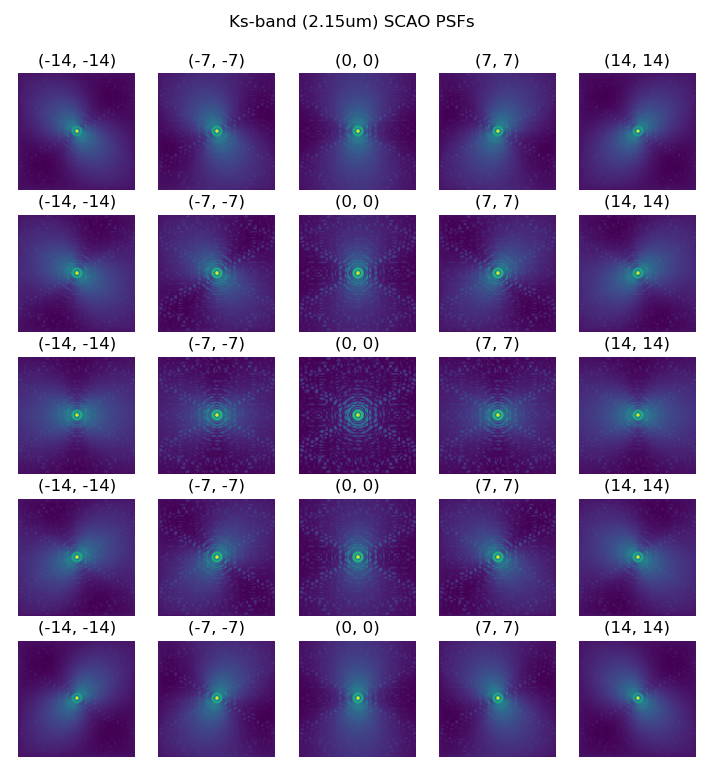AnisoCADO is the python package created around Eric Gendron’s code for analytically generating field-varying SCAO PSFs for the ELT.A grid of SCAO PSFs at 2.15um covering 14 arcsec on the MICADO field of view

# Installation¶

pip install anisocado


# Basic Usage¶

Note

See Getting Started for a more in-depth introduction

Warning

This is still the alpha release of AnisoCADO.

We will attempt to maintain backwards compatiblity, however we cannot guarantee that the API will remain the same as the package evolves.

The most needed functionality is based around the AnalyticalScaoPsf class. Create one like this

from anisocado import AnalyticalScaoPsf
psf = AnalyticalScaoPsf(N=512, wavelength=2.15)     # wavelength in um


where (for the moment) N is the side length of the PSF kernel image and wavelength is the central wavelength [um] of the PSF that we wish to simulate.When we create an AnalyticalScaoPsf object, an initial PSF is created that is on-axis. This can be accessed with the .psf_on_axis attribute.

To “move” the PSF off-axis, we call the .shift_off_axis(dx, dy) method. Here dx, dy are in arcseconds.

psf.shift_off_axis(15, -10)We can access this PSF in two ways: as a numpy array with .kernel or as an astropy ImageHDU object with .hdu. Here the kernel is kept in the .data attribute, while the header contains all the parameters used to create the PSF kernel:

psf.kernel
psf.hdu.data


## Write PSF to a FITS file¶

Given that the PSF can create an astropy ImageHDU object, we can take advantage of the astropy functionality and and override the .writeto() method of an astropy ImageHDU object:

psf.writeto("My_SCAO_PSF.fits")


Obviously this will only work for single PSFs. We will normally want to create multiple SCAO PSFs for different wavelengths and different positions over the field of view. To do this we can simply loop over a series of coordinates and add the HDUs to an astropy HDUList object.

from astropy.io import fits

psf = AnalyticalScaoPsf(N=256, wavelength=2.15)    # um
hdus = []
for x in np.arange(-25, 26, 12.5):
for y in np.arange(-25, 26, 12.5):
psf.shift_off_axis(x, y)
hdus += [psf.hdu]

hdu_list = fits.HDUList(hdus)
hdu_list.writeto("My_bunch_of_SCAO_PSFs.fits")# Dependencies¶

The following packages are required for normal operation:

• numpy (>=1.17)
• astropy

The following packages are optional:

• matplotlib

# Change Log¶

• 2021.10.21 :
• Upgraded Numpy dependency to v1.17 to use numpy.random.default_rng
• Removed support for Python 2.7